# 10.8 Vectors  (Page 10/22)

 Page 10 / 22

Suppose a body has a force of 3 pounds acting on it to the left, 4 pounds acting on it upward, and 2 pounds acting on it 30° from the horizontal. What single force is needed to produce a state of equilibrium on the body? Draw the vector.

5.1583 pounds, 75.8° from the horizontal

## Non-right Triangles: Law of Sines

For the following exercises, assume $\text{\hspace{0.17em}}\alpha \text{\hspace{0.17em}}$ is opposite side $\text{\hspace{0.17em}}a,\beta \text{\hspace{0.17em}}$ is opposite side $\text{\hspace{0.17em}}b,\text{\hspace{0.17em}}$ and $\text{\hspace{0.17em}}\gamma \text{\hspace{0.17em}}$ is opposite side $\text{\hspace{0.17em}}c.\text{\hspace{0.17em}}$ Solve each triangle, if possible. Round each answer to the nearest tenth.

$\beta =50°,a=105,b=45$

Not possible

$\alpha =43.1°,a=184.2,b=242.8$

Solve the triangle.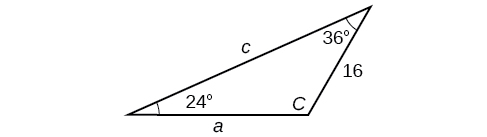$C=120°,a=23.1,c=34.1$

Find the area of the triangle.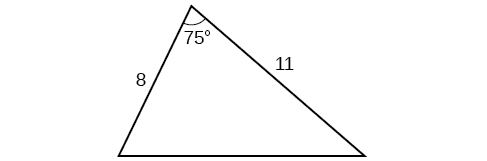A pilot is flying over a straight highway. He determines the angles of depression to two mileposts, 2.1 km apart, to be 25° and 49°, as shown in [link] . Find the distance of the plane from point $\text{\hspace{0.17em}}A\text{\hspace{0.17em}}$ and the elevation of the plane.

distance of the plane from point $\text{\hspace{0.17em}}A:\text{\hspace{0.17em}}$ 2.2 km, elevation of the plane: 1.6 km

## Non-right Triangles: Law of Cosines

Solve the triangle, rounding to the nearest tenth, assuming $\text{\hspace{0.17em}}\alpha \text{\hspace{0.17em}}$ is opposite side $\text{\hspace{0.17em}}a,\beta \text{\hspace{0.17em}}$ is opposite side $\text{\hspace{0.17em}}b,\text{\hspace{0.17em}}$ and $\text{\hspace{0.17em}}\gamma \text{\hspace{0.17em}}$ s opposite side

Solve the triangle in [link] , rounding to the nearest tenth.

$B=71.0°,C=55.0°,a=12.8$

Find the area of a triangle with sides of length 8.3, 6.6, and 9.1.

To find the distance between two cities, a satellite calculates the distances and angle shown in [link] (not to scale). Find the distance between the cities. Round answers to the nearest tenth.

40.6 km

## Polar Coordinates

Plot the point with polar coordinates $\text{\hspace{0.17em}}\left(3,\frac{\pi }{6}\right).$

Plot the point with polar coordinates $\text{\hspace{0.17em}}\left(5,-\frac{2\pi }{3}\right)$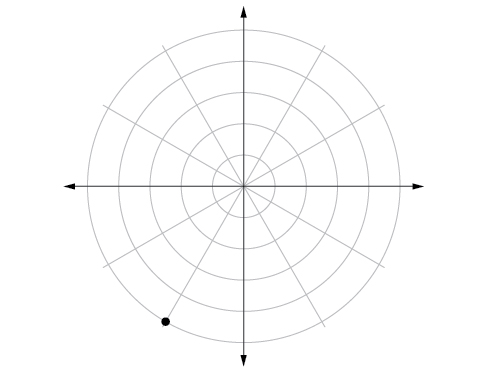Convert $\text{\hspace{0.17em}}\left(6,-\frac{3\pi }{4}\right)\text{\hspace{0.17em}}$ to rectangular coordinates.

Convert $\text{\hspace{0.17em}}\left(-2,\frac{3\pi }{2}\right)\text{\hspace{0.17em}}$ to rectangular coordinates.

$\text{\hspace{0.17em}}\left(0,2\right)\text{\hspace{0.17em}}$

Convert $\left(7,-2\right)$ to polar coordinates.

Convert $\left(-9,-4\right)$ to polar coordinates.

$\left(9.8489,203.96°\right)$

For the following exercises, convert the given Cartesian equation to a polar equation.

$x=-2$

${x}^{2}+{y}^{2}=64$

$r=8$

${x}^{2}+{y}^{2}=-2y$

For the following exercises, convert the given polar equation to a Cartesian equation.

$r=7\text{cos}\text{\hspace{0.17em}}\theta$

${x}^{2}+{y}^{2}=7x$

$r=\frac{-2}{4\mathrm{cos}\text{\hspace{0.17em}}\theta +\mathrm{sin}\text{\hspace{0.17em}}\theta }$

For the following exercises, convert to rectangular form and graph.

$\theta =\frac{3\pi }{4}$

$y=-x$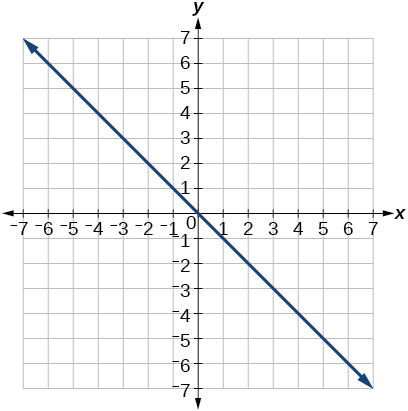$r=5\mathrm{sec}\text{\hspace{0.17em}}\theta$

## Polar Coordinates: Graphs

For the following exercises, test each equation for symmetry.

$r=4+4\mathrm{sin}\text{\hspace{0.17em}}\theta$

symmetric with respect to the line $\theta =\frac{\pi }{2}$

$r=7$

Sketch a graph of the polar equation $\text{\hspace{0.17em}}r=1-5\mathrm{sin}\text{\hspace{0.17em}}\theta .\text{\hspace{0.17em}}$ Label the axis intercepts.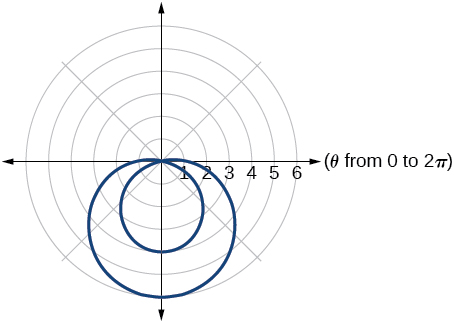Sketch a graph of the polar equation $\text{\hspace{0.17em}}r=5\mathrm{sin}\left(7\theta \right).$

Sketch a graph of the polar equation $\text{\hspace{0.17em}}r=3-3\mathrm{cos}\text{\hspace{0.17em}}\theta$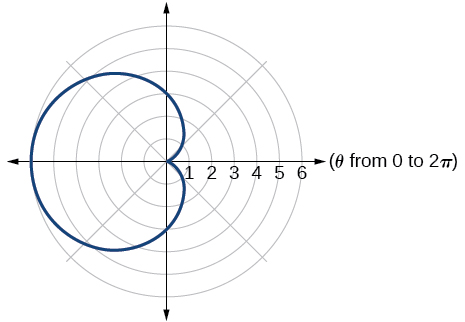## Polar Form of Complex Numbers

For the following exercises, find the absolute value of each complex number.

$-2+6i$

$4-\text{​}3i$

5

Write the complex number in polar form.

$5+9i$

$\frac{1}{2}-\frac{\sqrt{3}}{2}\text{​}i$

$\mathrm{cis}\left(-\frac{\pi }{3}\right)$

For the following exercises, convert the complex number from polar to rectangular form.

$z=5\mathrm{cis}\left(\frac{5\pi }{6}\right)$

$z=3\mathrm{cis}\left(40°\right)$

$2.3+1.9i$

For the following exercises, find the product $\text{\hspace{0.17em}}{z}_{1}{z}_{2}\text{\hspace{0.17em}}$ in polar form.

${z}_{1}=2\mathrm{cis}\left(89°\right)$

${z}_{2}=5\mathrm{cis}\left(23°\right)$

${z}_{1}=10\mathrm{cis}\left(\frac{\pi }{6}\right)$

${z}_{2}=6\mathrm{cis}\left(\frac{\pi }{3}\right)$

$60\mathrm{cis}\left(\frac{\pi }{2}\right)$

For the following exercises, find the quotient $\text{\hspace{0.17em}}\frac{{z}_{1}}{{z}_{2}}\text{\hspace{0.17em}}$ in polar form.

${z}_{1}=12\mathrm{cis}\left(55°\right)$

${z}_{2}=3\mathrm{cis}\left(18°\right)$

${z}_{1}=27\mathrm{cis}\left(\frac{5\pi }{3}\right)$

${z}_{2}=9\mathrm{cis}\left(\frac{\pi }{3}\right)$

$3\mathrm{cis}\left(\frac{4\pi }{3}\right)$

For the following exercises, find the powers of each complex number in polar form.

Find $\text{\hspace{0.17em}}{z}^{4}\text{\hspace{0.17em}}$ when $\text{\hspace{0.17em}}z=2\mathrm{cis}\left(70°\right)$

Find $\text{\hspace{0.17em}}{z}^{2}\text{\hspace{0.17em}}$ when $\text{\hspace{0.17em}}z=5\mathrm{cis}\left(\frac{3\pi }{4}\right)$

$25\mathrm{cis}\left(\frac{3\pi }{2}\right)$

#### Questions & Answers

The sequence is {1,-1,1-1.....} has
amit Reply
circular region of radious
Kainat Reply
how can we solve this problem
Joel Reply
Sin(A+B) = sinBcosA+cosBsinA
Eseka Reply
Prove it
Eseka
Please prove it
Eseka
hi
Joel
June needs 45 gallons of punch. 2 different coolers. Bigger cooler is 5 times as large as smaller cooler. How many gallons in each cooler?
Arleathia Reply
find the sum of 28th term of the AP 3+10+17+---------
Prince Reply
I think you should say "28 terms" instead of "28th term"
Vedant
if sequence sn is a such that sn>0 for all n and lim sn=0than prove that lim (s1 s2............ sn) ke hole power n =n
SANDESH Reply
write down the polynomial function with root 1/3,2,-3 with solution
Gift Reply
if A and B are subspaces of V prove that (A+B)/B=A/(A-B)
Pream Reply
write down the value of each of the following in surd form a)cos(-65°) b)sin(-180°)c)tan(225°)d)tan(135°)
Oroke Reply
Prove that (sinA/1-cosA - 1-cosA/sinA) (cosA/1-sinA - 1-sinA/cosA) = 4
kiruba Reply
what is the answer to dividing negative index
Morosi Reply
In a triangle ABC prove that. (b+c)cosA+(c+a)cosB+(a+b)cisC=a+b+c.
Shivam Reply
give me the waec 2019 questions
Aaron Reply
the polar co-ordinate of the point (-1, -1)
Sumit Reply

### Read also:

#### Get the best Algebra and trigonometry course in your pocket!

Source:  OpenStax, Algebra and trigonometry. OpenStax CNX. Nov 14, 2016 Download for free at https://legacy.cnx.org/content/col11758/1.6
Google Play and the Google Play logo are trademarks of Google Inc.

Notification Switch

Would you like to follow the 'Algebra and trigonometry' conversation and receive update notifications?ByByByBy Rhodes